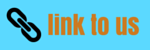# Linear Momentum

## Solved examples

Question 1 .A 1 kg ball moving at 12 m/s collides head on with 2 kg ball moving with 24 m/s in opposite direction.What are the velocities after collision if e=2/3?
a. v1=-28 m/s,v2=-4 m/s
b. v1=-4 m/s,v2=-28 m/s
c. v1=28 m/s,v2=4 m/s
d. v1=4 m/s,v2=28 m/s

Solution 1

Let v1 and v2 be the final velocities of the mass

Since the linear momentum is conserved in the collision
Momentum before =Momentum after
1*12+2*-24=1*v1+2*v2
Which becomes
-36=v1+2v2 ----1

Now
e=(v2 -v1)/(u1 -u2)

or 2/3= (v2 -v1)/[12-(-24)]
or
v2 -v1=24 ----2

Solving 1 and 2

v2=-4
v1=-28

Hence a is correct

Question 2.A moving bullet hits a solid target resting on a frictionless surface and get embeded in it.What is conserved in it?
a. Momentum Alone
b KE alone
c. Both Momentum and KE
d. Neither KE nor momentum

Solution 2 Since no external force is present,Momentum is conserved in the collision
Since the collison is in elastic ,KE is not conserved

Question 3. A stationary body of mass 3 kg explodes into three equal parts.Two of the pieces fly off at right angles to each other with the velocities 2i m/s and 3j m/s.If the explosion takes place in 10-3 sec.find out the average force action on the third piece in N
a.(-2i-3j)103
b. (2i+3j)103
c (2i-3j)10-3
d. none of these

Solution 3.

Net momentum before explosion zero
Since momentum is conserved in explosion
Net momentum after collosion is zero

Momentum of first part after explosion=2i
Momentum of second part after explosion=3j

So momentum of third part after explosion=-(2i+3j) as net momentum is zero

Now Net change is momentum of this part =-(2i+3j)
Now we know that
Average force X time =Net change in momentum
Average force=-(2i+3j) 103

hence a is correct

Question 4.A bullet of mass m is fired horizontally with a velocity u on a wooden block of Mass M suspended from a support and get embeded into it.The KE of th wooden + block system after the collisson
a.m2u2/2(M+m)
b.mu2/2
c. (m+M)u2/2
d. mMu2/2(M+m)

b>Solution 4.

Intial velocity of bullet=u
Intial velocity of block=0
So net momentum before collison=mu

Let v be the velocity after collision
Then Net momentum after collision=(M+m)v

Now linear momentum is conserved in this collision
so
mu=(M+m)v
or v=mu/(M+m)

So kinetic energy after collision
=(1/2)m2u2/2(M+m)

Hence a is correct

Question 5.A body of Mass M and having momentum p is moving on rough horizontal surface.If it is stopped in distance s.Find the value of coefficient of friction
a.p2/2M2gs
b. p/2Mgs
c. p2/2Mgs
d. p/2M2gs

Solution 5.

Deceleration due to friction=μg

Intial velocity=P/M

Now v2=u2 -2as
as v=0
P2/M2=2μgs
or μ=P2/2gsM2

Hence a is correct

Question 6.A rockets works on the principle of conservation of
a. Linear momentum
b.mass
c.energy
d. angular momentum

Solution 6. A rocket works on the principle of linear momentum.

Question 7.A flat car of weight W roll without resistance along on a horizontal track .Intially the car together with weight w is moving to the right with speed v.What invcrement of the velocity car will obtain if man runs with speed u reltaive to the floor of the car and jumps of at the left?
a.wu/w+W
b. Wu/W+w
c. (W+w)u/w
d. none of the above

Solution 7 Considering velocities to the right as positive
The intial momentum of the system is
=[(W+w)/g]v

Let Δv be the increment in velocity then

Final momentun of the car is
(W/g)(v+Δv)

While that of man is
(w/g)(v+Δv-v)

Since no external forces act on the system,the law of conservation of momentum gives then
[(W+w)/g]v=(W/g)(v+Δv)+(w/g)(v+Δv-v
or Δv=wu/(W+w)

Question 8.Consider the following two statements.
STATEMENT 1 Linear momentum of a system of particles is zero.
STATEMENT 2 Kinetic energy of system of particles is zero.
(A) A does not imply B and B does not imply A.
(B) A implies B but B does not imply A
(C) A does not imply B but b implies A�
(D) A implies B and B implies A.

Solution 8
Net momentum=m1v1+m2v2
Net Kinectic Energy=(1/2)m1v12+(1/2)m2v22

Let v1=v ,v2=-v and m1=m2=m
Then Net momentum=0 but Net Kinectic Energy is not equal to zero

Now lets v1= v2=0

Then Net Kinectic Energy=0 and Net momentum=0
Hence (c) is correct